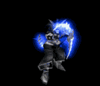• 0 Vote(s) - 0 Average
• 1
• 2
• 3
• 4
• 5
 Spell checker with R
25-02-2014, 12:34 PM,Post: #1TimonTBD MembersPosts: 167 Joined: Jul 2011 Reputation: 0Spell checker with R
Hello all saja nk share buat spell checker dari peter norvig dengan R dengan dictionarynya
asalnye R nie statistical language untuk buat charts dengan grahs according to data x sesuai buat logical programming but... fuk da police right ?Code:
```words <- scan("http://norvig.com/big.txt", what = character()) counts <- table(words) # This is a text processing function, which I # borrowed from a CMU Data mining course professor. strip.text <- function(txt) {   # remove apostrophes (so "don't" -> "dont", "Jane's" -> "Janes", etc.)   txt <- gsub("'","",txt)   # convert to lowercase   txt <- tolower(txt)   # change other non-alphanumeric characters to spaces   txt <- gsub("[^a-z0-9]"," ",txt)   # change digits to #   txt <- gsub("[0-9]+"," ",txt)   # split and make one vector   txt <- unlist(strsplit(txt," "))   # remove empty words   txt <- txt[txt != ""]   return(txt) } # Words within 1 transposition. Transpositions <- function(word = FALSE) {   N <- nchar(word)   if (N > 2) {     out <- rep(word, N - 1)     word <- unlist(strsplit(word, NULL))     # Permutations of the letters     perms <- matrix(c(1:(N - 1), 2:N), ncol = 2)     reversed <- perms[, 2:1]     trans.words <- matrix(rep(word, N - 1), byrow = TRUE, nrow = N - 1)     for(i in 1:(N - 1)) {       trans.words[i, perms[i, ]] <- trans.words[i, reversed[i, ]]       out[i] <- paste(trans.words[i, ], collapse = "")     }   }   else if (N == 2) {     out <- paste(word[2:1], collapse = "")   }   else {     out <- paste(word, collapse = "")   }   return(out) } # Single letter deletions. Deletes <- function(word = FALSE) {   N <- nchar(word)   out<-mat.or.vec(1,N)   word <- unlist(strsplit(word, NULL))   for(i in 1:N) {     out[i] <- paste(word[-i], collapse = "")      }   return(out) } # Single-letter insertions. Insertions <- function(word = FALSE) {   N <- nchar(word)   out <- list()   for (letter in letters) {     out[[letter]] <- rep(word, N + 1)     for (i in 1:(N + 1)) {       out[[letter]][i] <- paste(substr(word, i - N, i - 1), letter,                                 substr(word, i, N), sep = "")        }   }   out <- unlist(out)   return(out) } # Single-letter replacements.      Replaces <- function(word = FALSE) {      N <- nchar(word)   out <- list()   for (letter in letters) {     out[[letter]] <- rep(word, N)     for (i in 1:N) {       out[[letter]][i] <- paste(substr(word, i - N, i - 1), letter,                                 substr(word, i + 1, N + 1), sep = "")          }   }   out <- unlist(out)   return(out) } # All Neighbors with distance "1" Neighbors <- function(word) {   neighbors <- c(word, Replaces(word), Deletes(word),                  Insertions(word), Transpositions(word))   return(neighbors) } # Probability as determined by our corpus. Probability <- function(word, dtm) {   # Number of words, total   N <- length(dtm)   word.number <- which(names(dtm) == word)   count <- dtm[word.number]   pval <- count/N   return(pval) } # Correct a single word. Correct <- function(word, dtm) {   neighbors <- Neighbors(word)   # If it is a word, just return it.   if (word %in% names(dtm)) {     out <- word   }   # Otherwise, check for neighbors.   else {     # Which of the neighbors are known words?     known <- which(neighbors %in% names(dtm))     N.known <- length(known)     # If there are no known neighbors, including the word,     # look farther away.     if (N.known == 0) {       print(paste("Having a hard time matching '", word, "'...", sep = ""))       neighbors <- unlist(lapply(neighbors, Neighbors))     }     #Then out non-words.     neighbors <- neighbors[which(neighbors %in% names(dtm))]     N <- length(neighbors)     # If we found some neighbors, find the one with the highest     # p-value.     if (N >= 1) {       P <- 0*(1:N)       for (i in 1:N) {         P[i] <- Probability(neighbors[i], dtm)       }       out <- neighbors[which.max(P)]     }     # If no neighbors still, return the word.     else {       out <- word     }      }      print(out)   distance <- stringdistmatrix(out,word,"dl")      print(distance) } # Correct an entire document. CorrectDocument <- function(document, dtm) {   by.word <- unlist(strsplit(document, " "))   N <- length(by.word)   for (i in 1:N) {     by.word[i] <- Correct(by.word[i], dtm = dtm)   }    distance <- stringdistmatrix(corrected,document,"dl")   corrected <- paste(by.word, collapse = " ")   return(corrected)   print(distance)```
ini hasil dari peter norvig tetapi kita bole improve accuracy nye dengan menukar edit functionsnya dengan iniCode:
```library(plyr) Insert_Vowels<- function(word = FALSE) {    N <- nchar(word)    out <- list()    data<-laply(seq(1,nchar(word),1), function(i) substr(word, i, i))    yesno<-is.element(data,vowels)    position<-which(yesno==TRUE)      for (letter in vowels) {     out[[letter]] <- rep(word, length(position))              for( i in 1:length(position))     {      item<-out[[letter]][i]      data2<-laply(seq(1,nchar(item),1), function(i) substr(item, i, i))      answer<-paste(substr(item,0,position[i]),vowels, substr(item,position[i]+1,N),sep="")      answer2<-paste(substr(item,0,position[i]-1),vowels, substr(item,position[i],N),sep="")      print(answer)      print(answer2)        }    out <- unlist(out)   return(out) } } Insert_Vowels("aeroplane")```

dan
Code:
```library(plyr) Insert_Vowels<- function(word = FALSE) {    N <- nchar(word)    out <- list()    data<-laply(seq(1,nchar(word),1), function(i) substr(word, i, i))    yesno<-is.element(data,vowels)    position<-which(yesno==TRUE)      for (letter in vowels) {     out[[letter]] <- rep(word, length(position))              for( i in 1:length(position))     {      item<-out[[letter]][i]      data2<-laply(seq(1,nchar(item),1), function(i) substr(item, i, i))      answer<-paste(substr(item,0,position[i]),vowels, substr(item,position[i]+1,N),sep="")      answer2<-paste(substr(item,0,position[i]-1),vowels, substr(item,position[i],N),sep="")      print(answer)        }    out <- unlist(out)   return(out) } } Insert_Vowels("awesome")```

nk explain susah skang kt tmpt keja nnty explain len ek ?

toodles25-02-2014, 12:35 PM,Post: #2lamkiTBD Unregistered UserPosts: 226 Joined: May 2011 Reputation: 2RE: Spell checker with R
macamana nak tun R language nim ~Hmm
Revenge 101 :
Spoiler:

1.Pee in plate
2.Freeze the pee(in 1.)
3.Slide frozen 'pee disc' under the victim's door(room or house, any will do)
4.The pee disc will now melt.
5.???
6.Profit25-02-2014, 12:38 PM,Post: #3TimonTBD MembersPosts: 167 Joined: Jul 2011 Reputation: 0RE: Spell checker with R
(25-02-2014, 12:35 PM)lamki Wrote: macamana nak tun R language nim ~Hmm

nak tun R language ?wot ?« Next Oldest | Next Newest »

Forum Jump: